### Structured SVM and Structured Perceptron for CRF learning in Python

[EDIT: If you are reading this now, have a look at pystruct.github.io. The project matured quit a bit in the meantime.]

Today I pushed some of my code to github that I use for experimenting with CRF learning. This goes along the lines of my recent posts on graphcut and I hope to post a full CRF learning framework for semantic image segmentation soon. This is a pretty standard setup in computer vision, but I really haven't found much code online.

Actually I haven't found any code to learn loopy CRFs, so I hope my simple implementation can help to get a better understanding of these methods. It certainly helped me ;)

Let me start by saying how a structured perceptron works.
Given a training set (x^i, y^i), a structured perceptron tries to find a function f such that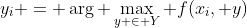This means is basically tries to minimize the zero-one loss.

The function f is given by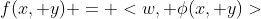so it is a linear function of some joint feature phi of x and y parametrized by w.
Learning basically uses the perceptron algorithm on phi. One iteration of learning does:

For each example (x^i, y^i):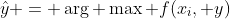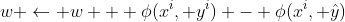This is very easy to implement and already gives nice results in many cases.
So how do we apply this to CRF learning?
For ease of exposition, assume a binary CRF. The parameters are pairwise and unary potentials, usually written like this: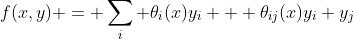So for using a structured perceptron for CRF learning, we basically need to put these theta in the w above and we need to find the argmax somehow.
In my implementation, the argmax is done using QPBO (my Python bindings are here and work the same as my gco bindings). This is a graph-cut based inference procedure for general energy functions. Another possiblity would be to use TRW-S.

I like to use graph-cut for inference since it is usually much faster than message passing. The problem is, that the usual methods only work with submodular energy functions. If you do learning, you would need to restrict yourself to these kind of functions - which I'm not doing here.

I implemented some very simple forms of CRFs, but they should be easy enough to extend. I am using global parameters theta and the unary terms are just given by a scalar weighting of x.
So this is more of a traditional MRF with learned affinities.

Here are some simple examples included in my code.
Learning to denoise blocks:
Learning a checkerboard (this is sort of interesting since a non-submodular function is learned:

Learning to denoise stripes:
These are obviously very simple examples but I feel the still convey the spirit of the method.

My code also includes a very naive Structured SVM.
The details of learning a structure SVM are well described in Tsochantaridis, T. Joachims, T. Hofmann, and Y. Altun Large Margin Methods for Structured and Interdependent Output Variables and I won't go into the details here.
Similar to the structured perceptron, it alternates two steps: the argmax and the update.
The update here is solving the dual formulation of the SVM-version of the linear function described above, where constraints are derived from the "negative examples" obtained by the argmax.
I solve this dual formulation simply using cvxopt.
I say my implementation is pretty naive since I optimize the dual from scratch each time I add new constraints. Since I am mainly interested in learning for loopy CRFs where inference is hard, this doesn't matter so much, as solving the optimization is much faster than doing the inference.
Any hints on how to do this better are still welcome ;)

There is one more significant difference between the structured perceptron and the structured SVM: to obtain the constraints (="negatives") in the SVM formulation, one needs to take your loss into account - for CRFs usually the number of variables that are labeled wrong. This is the so-called "loss augmented prediction". The idea is that not only should all negatives have lower energy as the ground truth, they should also have a margin that is as big as their loss. (This is the slack-rescaled version which I implemented).
This can be done by modifying the unary potentials basically by subtracting the energy of the ground truth.

For the simple examples above, the SVM doesn't give very different results to the Perceptron, so I won't show the images.
I hope this is useful and I'd love to get some feedback and suggestions.

[update] I forgot to mention that my toy CRF implementation not only works on grid graphs. There is also a version for general graphs.[/update]

### Comments

1.Out of curiosity, what problem are you personally trying to solve, if any? It is difficult for me to get involved in these sorts of areas without a concrete problem to solve.

1.There is a lab report presented in the Lisbon Machine Learning school. The application problem lies in the natural language processing. Have a look at.

2.http://lxmls.it.pt/2011/guide_day3.pdf

2.The application I am working on is semantic image segmentation. The idea is to segement an image into a fixed set of semantic object classes. In my application, the nodes will not represent pixels but superpixels (like these http://peekaboo-vision.blogspot.de/2012/05/superpixels-for-python-pretty-slic.html).
If you are interested, here is a relative recent paper on the subject: http://kev-smith.com/papers/LUCCHI_ICCV11.pdf